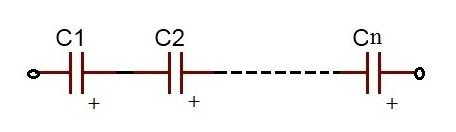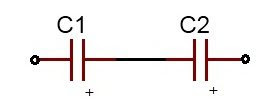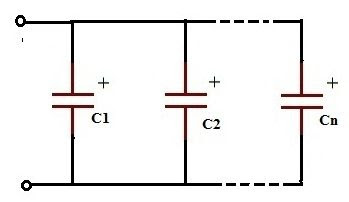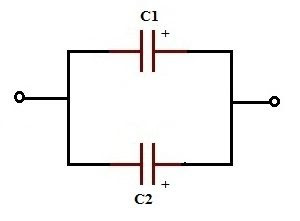# Capacitors in Series and Parallel:

Capacitor in Series:

Capacitors in series meanstwo or more capacitors are connected in a single linei.e positive plate of the one capacitor is connected to the negative plate of the next capacitor. All the capacitors in series have equal charge (Q) and equal charging current (iC).
Consider N- numbers of capacitors are connected in series, then
QT =Q1 = Q2 = Q3 = ———- = QN
IC = I1 = I2 = I3 = ——— = IN

### Capacitors in a Series Connection:

The following circuits show the series connection of group of capacitors.
series connection of N-number of capacitors.series connection of two capacitors.In this circuit the charge (Q) stored in all capacitors is same because every capacitor has the charge which is flowing from the adjacent capacitor. The voltage drop in all capacitors is different from each other. But the total voltage drop applied between input and output lines of the circuit is equal to the sum of all the individual voltage drops of each capacitor. The equivalent capacitance of the circuit is Ceq = Q/V.
Thus,
VT = V1 + V2
Ceq = Q/V1 + Q/V2
1/Ceq = (V1+ V2)/Q
VT = Q/Ceq = Q/C1 + Q/C2

### Series Capacitors Equation:

1/Ceq = 1/C1 + 1/C2 +……… + 1/CN
When the capacitors are in series connection the reciprocal of the equivalent capacitance is equal to the sum of the reciprocals of the individual capacitances of the capacitors in the circuit.
From the figure 2, the reciprocal of equivalent capacitance value of the circuit is equal to the sum of reciprocal capacitances values of two capacitors C1 and C2, the expression is given below.
1/Ceq = 1/C1 + 1/C2
Capacitors in Series - Example No1:
We assume that the values of two capacitors in the above figure2 are 0.4uF and 0.5uF respectively. Now we can calculate the equivalent capacitance for the two capacitors in series as,
1/Ceq = 1/C1 + 1/C2
1/Ceq = (C1 + C2)/ C1C2
Ceq = (C1C2) / (C1 + C2)
Ceq = (0.4uF*0.5uF) / (0.4uF+0.5uF)
Ceq = 0.22uF
We know that the equivalent capacitance of the capacitors in series connection is less than the value of the smallest capacitor in the circuit. In that way here also we get the equivalent value 0.22 uF is less than the smallest capacitance 0.4 uF in the series connection of the given two capacitors circuit.

### Capacitors in Series - Example No2:

Calculate the equivalent capacitance and the individual voltage drops across the set of two capacitors in series have 0.1uF and 0.2uF respectively when connected to a 12V a.c. supply.
Equivalent capacitance,
1/Ceq = 1/C1 + 1/C2
Ceq = (C1C2) / (C1 + C2)
Ceq = (0.1uF*0.2uF) / (0.1uF+0.2uF)
Ceq = 0.066uF = 66nF
Voltage drops across the two given capacitors in series are,
V1 = (C2*VT)/ (C1+C2) = (0.2uF*12V)/ (0.1uF+0.2uF) = 8Volts
V2 = (C1*VT)/ (C1+C2) = (0.1uF*12V)/ (0.1uF+0.2uF) = 4Volts
From these results we observed that the equivalent capacitance 66nF is less than the smallest capacitance 0.1uF of the given two capacitors. The individual voltage drops across the given two capacitors are different. But the sum of individual voltage drops of both the capacitors is equal to the total voltage. i.e  8V+4V=12V.
Now we calculate the charge stored in individual capacitor,
Q1 = V1* C1 = 8V * 0.1uF = 0.8uC
Q2 = V2* C2 = 4V * 0.2uF = 0.8uC
Here we observed that equal charge 0.8uC is stored in both the capacitors C1 and C2 which are connected in series.

### Capacitors in Series Summary:

The equivalent capacitance of the capacitors connected in series chain is, the reciprocal of the equivalent capacitance is equal to the sum of the reciprocals of all the individual capacitances of the capacitors connected in series. All the capacitors which are connected in series have the equal charge (Q). The charging current ( iC) is also same for all the individual capacitors which are connected in series like iC=i1= i2 etc.
The voltage drops for individual capacitors in series connection are different. But the sum of all the individual voltage drops is equal to the total voltage across the circuit. i.e.VT = V1+V2 etc . A large value of capacitance will result in a smaller voltage drop while a small capacitance value will result in a larger voltage drop.

### Capacitors in Parallel Circuits:

Capacitors in parallel means two or more capacitors are connected in parallel way, i.e. both of their terminals are connected to each terminal of the other capacitor or capacitors respectively. All the capacitors which are connected in parallel have the same voltage and is equal to the VT applied between the input and output terminals of the circuit. Then, parallel capacitors have a ‘common voltage’ supply across them .i.e. VT = V1 = V2 etc.
The equivalent capacitance, Ceq of the circuit where the capacitors are connected in parallel is equal to the sum of all the individual capacitances of the capacitors added together. This is because the top plateof each capacitor in the circuit is connected to the top plate of adjacent capacitors. In the same way the bottom plate of each capacitor in the circuit is connected to the bottom plate of adjacent capacitors.
The following circuits show parallel connection between groups of capacitors. Figure3 has N-number of capacitors and figure 4 has two capacitors connected in parallel.Parallel connection of two capacitors,In  above figure  the total charge (Q) across the circuit is divided between the two capacitors, means the charge Q distributes itself between the capacitors connected in parallel. Because the voltage drop across individual capacitors is equal and also it is equal to the total voltage applied to the circuit. But the total charge Q is equal to the sum of all the individual capacitor charges connected in parallel. i.e. From the above figure the two different capacitors C1 and C2 have two different charges Q1 and Q2 respectively. Here Q=Q1+Q2
Now we see the equivalent capacitance of the capacitors C1 and C2 connected in parallel which shown in  the above figure.
We know the formula,
Q=Ceq VT
Here, Q = Q1+Q2
And VT = V1 = V2
Ceq=Q/VT = (Q1+Q2)/VT = (Q1/VT) + (Q2/VT)

### Parallel Capacitors Equation:

Ceq = C1+C2+C3+ ———— +CN
The equivalent capacitance of the capacitors which are connected in parallel is equal to the sum of the individual capacitances of the capacitors in the circuit.
From the figure 4, the equivalent capacitance (Ceq) value is equal to the sum of both the capacitance values of C1 and C2, the expression is shown below.
Ceq = C1+C2

### Capacitors in Parallel - Example No1:

Consider the capacitance values of the two capacitors  C1 =0.2uF and C2 =0.3uF which are shown in above figure 4. Now calculate the equivalent capacitance of the circuit.
We know that the Equivalent capacitance,
Ceq = C1 + C2
Ceq =0.2uF + 0.3uF
Ceq =0.5uF
One important point to remember about parallel connected capacitor circuits, the equivalent capacitance (Ceq) of any two or more capacitors connected together in parallel will always be greater than the value of the largest capacitor in the circuit as we are adding together values. So in our example above Ceq=0.5uF whereas the largest value capacitor in the circuit is only 0.3uF.

### Capacitors in Parallel - Example No2:

Calculate the equivalent capacitance across the set of two capacitors in parallel shown in figure 4.
(a) One  capacitor of 0.1uF connected in parallel with 0.2uF.
(b) One  capacitor of 750 nF connected in parallel with 0.5 uF.
(a) Equivalent capacitance,
Ceq = C1 + C2
Ceq =0.1uF + 0.2uF
Ceq =0.3uF
(b) Equivalent capacitance,
Ceq = C1 + C2
Ceq =750nF + 0.5uF
Ceq =750nF + 500nF
Ceq = 1250 nF =1.25uF​
In the above two cases we observed that the equivalent capacitance (Ceq) value of the two capacitors connected together in parallel is greater than the value of the largest capacitor in the circuit as we are adding together values. In section (a) the value of Ceq = 0.3uF whereas the largest value capacitor is only 0.2uF. In section (b) the value of Ceq= 1.25uF whereas the largest value capacitor in the circuit is 0.5uF only.

### Capacitors in parallel Summary:

The equivalent capacitance of the group of capacitors which are connected in parallel is equal to the sum of capacitances of the individual capacitors. i.e Ceq = C1+C2. Because the voltage drop across the individual capacitor is equal to the total voltage applied between the input and output terminals of the circuit. i.e. VT = V1=V2. The charges stored in individual capacitors are different but the sum of all the individual capacitor charges is equal to the total charge flows in the circuit. i.e. Q = Q1+Q2.
The charging current flow in circuit is distributed to all the capacitors in the circuit. But the total charging current is equal to the sum of all the individual charging currents of the capacitors in the circuit. i.e. iC=i1+i2 etc. The equivalent capacitance value of the group of capacitors which are connected in parallel is always greater than the value of the largest capacitor in the circuit.
REFERENCE // Electronics Hub